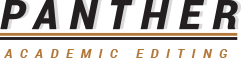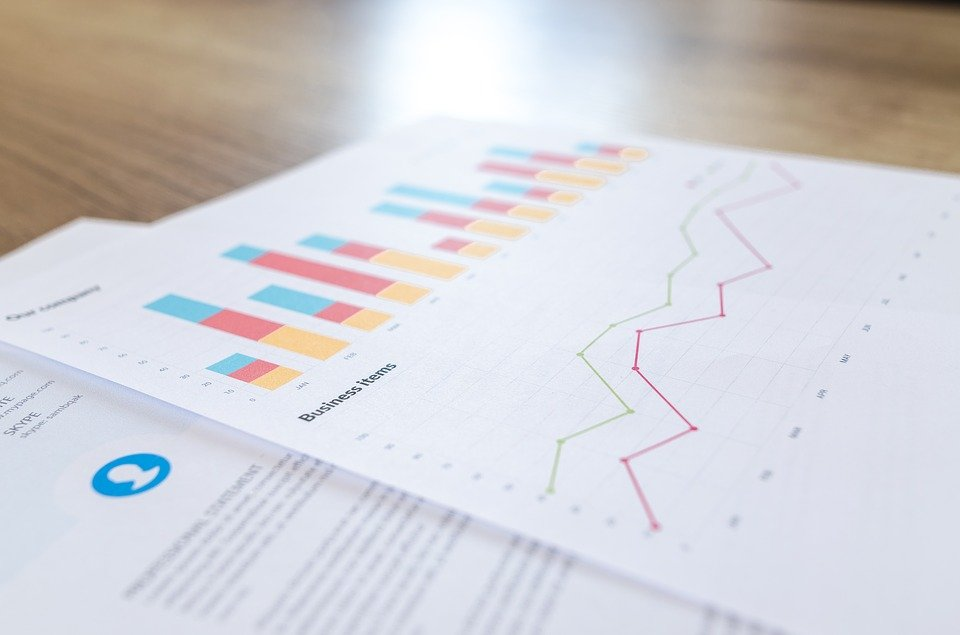Discussion –

0

Discussion –

0

# Research Statistics 101: A PrimerStatistics is a robust tool when carrying out data analysis and research. At a higher level, statistics can help you analyze large data sets. While a basic bar graph may overview a trend, statistics can allow us to use data in a targeted and information-driven way. Here are some basic statistical concepts used in research and analysis.

## 1.   Mean

In layman’s terms, mean is typically known as the average of a data set. It’s calculated by adding all the numbers in a list and then dividing the total sum by the number of items. Mean can come in handy when we have to determine the overarching trend in a data set. It can offer us a quick picture of a large data set. The best thing about mean is that it’s easy to calculate.

## 2.   Standard Deviation

Standard deviation measures how well the data is spread around the mean. The Greek letter sigma is used to represent it. If the standard deviation value is high, it means that the data is widely spread out from the mean. Low standard deviation refers to data that’s more in line with the mean’s numerical value. Standard deviation is essential in research as it can determine data dispersion quickly.

## 3.   Regression

Regression shows a relationship between explanatory and dependent variables, and it is represented through a scatterplot. A regression line shows whether this relationship is weak or strong. Concepts of regression are taught in college or high school level statistics with applications in business and science to determine different trends over time.

## 4.   Sample Size Determination

When you measure a large population or data set, like the women workforce in small scale businesses, you don’t have to gather data from every woman working in small scale businesses. A sample can do this job. You just have to determine the accurate size of the sample. Using standard deviation and proportion methods, you can accurately calculate the ideal sample size that you need to call your data statistically significant.

## 5.   Hypothesis Testing

Hypothesis testing is also known as t-testing. It can evaluate if a particular assumption about the population or data set is correct or not. In statistics and data analysis, you have to count a hypothesis test results as statistically significant if the same results couldn’t have been achieved through a random chance. From hard sciences to economics and business, all disciplines use hypothesis testing.